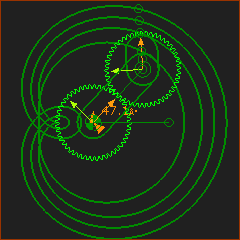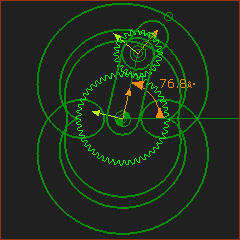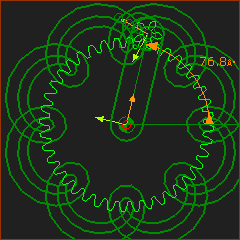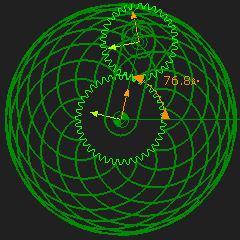﻿ Getting Started Tutorials - MechDesigner > Tutorial 14: Gear-Pairs / Rack-Pinions > B: Orbiting Gear Centres > Epictrochoid: Epicycloid, Contracted and Protracted

# Epictrochoid: Epicycloid, Contracted and Protracted

### B: ORBITING GEAR center

#### Epicycloid and Epitrochoid Curves

 Epicyclic Gears are arranged so that a gear rolls around the outside of a fixed gear. You can show a Trace-Point of a Point on the rolling gear. The curve is called an Epicycloid if the point is on the pitch-circle of the rolling gear. The curve is called an Epitrochoid if the point is not on the pitch-circle of the rolling gear. If the point is inside of the Pitch-Circle, it is called Contracted (also Curtate), and if outside the pitch-circle it is called Protracted (also Prolate). Epitrochoid Curves are traced by a Point on a gear that rolls (orbits) around the outside of the fixed internal gear. The shape of the curve depends on the ratio of the teeth. With gears, the ratio of the teeth is always a rational number. Therefore, the curve will always repeat, eventually, even if the gear ratio is a ratio of two prime numbers.Equal Number of Gear Teeth. This curve is called a Limaҫon. If the Point is on the Pitch-Circle it is called a Cardioid. The image shows four curves. Epicycloid One curve is by a Point on the pitch-circle of the orbiting gear - it is the Epicycloid Epitrochoid Prolate Epitrochoid curves are by points outside the pitch-circle. Curtate Epitrochoid curvesis given by a point inside the pitch-circle.The External Gear is half the diameter of the fixed gear. The image shows four curves. Epicycloid One curve is given by a point on the pitch-circle - it is the Epicycloid Epitrochoid Two curves are by points outside the pitch-circle . One curve is by a point inside the pitch-circle.The Gear ratio is 8: 1 There are three curves.